# Compressibility factor (gases)

The compressibility factor (Z) is a useful thermodynamic property for modifying the ideal gas law to account for behavior of real gases. It is a measure of how much the thermodynamic properties of a real gas deviate from those expected of an ideal gas. It may be thought of as the ratio of the actual volume of a real gas to the volume predicted by the ideal gas at the same temperature and pressure as the actual volume.

For an ideal gas, Z always has a value of 1. For real gases, the value may deviate positively or negatively, depending on the effect of the intermolecular forces of the gas. The closer a real gas is to its critical point or to its saturation point, the larger are the deviations of the gas from ideal behavior.

The upper graph in Figure 1 illustrates how the compressibility factor varies for different gases at the same temperature and pressure. The lower graph illustrates how the compressibility factor of a gas (for example, methane) at a given pressure varies with temperature.

This article deals only with the compressibility factor of gases and does not delve into the compressibility of liquids or vapor-liquid mixtures.

##  Determination of gas compressibility values

The ideal gas law is defined as:$P\,V_\mathrm{m} = R\,T$

and the ideal gas law corrected for non-ideality is defined is: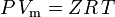$P\,V_\mathrm{m} = ZR\,T$
P where: = pressure = molar volume of the gas = compressibility factor = Universal gas constant = temperature

and thus:$Z = \frac{\;P\,V_\mathrm{m}}{R\,T}$

which is the simplest and most widely used real gas equation of state (EOS). The major limitation of this equation of state is that the gas compressibility factor, Z, is not a constant but varies from one gas to another as well as with the temperature and pressure of the gas under consideration. It must be determined experimentally.

Where experimental data is available for specific gases, that data may be used to produce graphs (such as in Figure 1) of Z versus pressure at a constant temperature or of Z versus pressure for various temperatures for those specific gases. Such graphs are useful for readily obtaining interpolated values of Z between the experimentally determined values.

The compressibility factor, as mentioned earlier, may also be expressed as: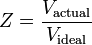$Z = \frac{V_\mathrm{actual}}{V_\mathrm{ideal}}$

There are three regimes that affect the compressibility factor:

1. the value of Z tends toward 1 as the gas pressure approaches 0, where all gases tend toward ideal behavior
2. the value of Z is less than 1 at intermediate pressures because the intermolecular forces of attraction cause the actual volumes to be less than the ideal values
3. the value of Z is greater than 1 and ultimately tends toward infinity at high pressures because the intermolecular repulsive forces cause the actual volumes to be greater than the ideal values

### Generalized compressibility factor graphs for pure gases

The unique relationship between the compressibility factor and the reduced temperature, Tr, and the reduced pressure, Pr, was first recognized by van der Waals in 1873 and is known as the two-parameter principle of corresponding states. The principle of corresponding states expresses the generalization that the properties of a gas which are dependent on intermolecular forces are related to the critical properties of the gas in a universal way. That provides a most important basis for developing correlations of molecular properties.

As for the compressibility of gases, the principle of corresponding states indicates that any pure gas at the same reduced temperature, Tr, and reduced pressure, Pr, should have the same compressibility factor. 

The reduced temperature and pressure are defined as:$T_r = \frac{T}{T_c}$   and$P_r = \frac{P}{P_c}$

Tc and Pc are known as the critical temperature and critical pressure of a gas. They are characteristics of each specific gas with Tc being the temperature above which it is not possible to liquify a given gas and Pc is the minimum pressure required to liquify a given gas at its critical temperature. Together they define the critical point of a fluid above which distinct liquid and gas phases of a given fluid do not exist.

The pressure-volume-temperature (PVT) data for real gases varies from one pure gas to another. However, when the compressibility factors of various single-component gases are graphed versus pressure along with temperature isotherms (as shown for methane in the lower graph of Figure 1), many of the graphs exhibit similar isotherm shapes.

In order to obtain a generalized graph that can be used for many different gases, the reduced pressure and temperature, Pr and Tr, are used to normalize the compressibility factor data. Figure 2 is an example of a generalized compressibility factor graph derived from hundreds of experimental P-V-T data points of 10 pure gases, namely methane, ethane, ethylene, propane, n-butane, i-pentane, n-hexane, nitrogen, carbon dioxide and steam.

There are more detailed generalized compressibility factor graphs based on as many as 25 or more different pure gases, such as the Nelson-Obert graphs. Such graphs are said to have an accuracy within 1-2 percent for Z values greater than 0.6 and within 4-6 percent for Z values of 0.3-0.6.

The generalized compressibility factor graphs may be considerably in error for strongly polar gases which are gases for which the centers of positive and negative charge do not coincide. In such cases the estimate for Z may be in error by as much as 15-20 percent.

The quantum gases hydrogen, helium, and neon do not conform to the corresponding-states behavior and the reduced pressure and temperature for those three gases should be redefined in the following manner to improve the accuracy of predicting their compressibility factors when using the generalized graphs: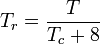$T_r = \frac{T}{T_c + 8}$   and$P_r = \frac{P}{P_c + 8}$

where the temperatures are in degrees Kelvin and the pressures are in atmospheres.

### Generalized compressibility factor graphs for gas mixtures

The generalized compressibility factor graphs for pure gases (such as exemplified in Figure 2 or the Nelson-Obert graphs may also be used for gas mixtures by using Kay's Rule proposed by W. B. Kay in 1936. That rule predicts the P-V-T behavior of a gas mixture by determining the compressibility factor for a gas mixture at the reduced temperature and reduced pressure defined in terms of the pseudocritical temperature, Tpc and the pseudocritical pressure, Ppc which are defined as: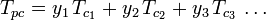$T_{pc} = y_1\,T_{c_1} + y_2\,T_{c_2} + y_3\,T_{c_3}\,\dots$$P_{pc} = y_1\,P_{c_1} + y_2\,P_{c_2} + y_3\,P_{c_3}\,\dots$
Tpc where: = the pseudocritical temperature of the gas mixture = the critical temperature of a component of the gas mixture = the pseudocritical pressure of the gas mixture = the critical temperature of a component of the gas = the mole (or volume) fraction of a component of the gas

The pseudoreduced temperature, Tpr, and pseudoreduced pressure, Ppr, to use with the generalized compressibility factor graphs for pure gases are then:$T_{pr} = \frac{T}{T_{pc}}$   and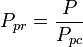$P_{pr} = \frac{P}{P_{pc}}$

A compressibility factor graph for natural gas (which is a mixture of hydrocarbon and other gases) using pseudoreduced temperatures and pressures is available on the Internet.

###  The van der Waals equation

The van der Waals equation was developed in 1873 and may be expressed as:$\left(P + \frac{a}{V_\mathrm{m}^2}\right)\left(V_\mathrm{m} - b\right) = R\,T$

where:

a is a measure of the strength of attraction between the gas molecules
b accounts for the volume occupied by gas molecules, which decreases the available open volume

The van der Waals equation may be re-arranged as:$P = \frac{R\,T}{V_\mathrm{m} - b} - \frac {a}{V_\mathrm{m}^2}$

and the compressibility factor can be written as:$Z = \frac{\;P\,V_\mathrm{m}}{R\,T} = \frac{V_\mathrm{m}}{R\,T}\left(\frac{R\,T}{V_\mathrm{m} - b} - \frac{a}{V_\mathrm{m}^2}\right)$

and we now have an equation for determining Z by using the van der Waals parameters a and b:$Z = \frac{V_\mathrm{m}}{V_\mathrm{m} - b} - \frac{a}{R\,T\,V_\mathrm{m}}$

Although a and b are referred to as "the Van der Waals constants", they are not truly constants because they vary from one gas to another; they are, however, independent of P, V and T. In other words, they are constant for the gas being considered. Given the critical temperature and pressure for a specific gas, a and b can be obtained for that specific gas from these equations: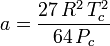$a = \frac{27\, R^2\, T_c^2}{64\, P_c}$   and$b = \frac{R\, T_c}{8\, P_c}$

For example, the critical pressure of ethane is 4.884 MPa and the critical temperature is 305.43 K (32.3˚C). Using the universal gas constant, R, of 8.3145 Pa m3 K-1 mol-1, the above equations yield a = 0.557 m6 Pa mol-2 and b = 6.500×10-5 m3 mol-1.

Tabulated values of a and b for various gases are available in these references as well as many other sources in the technical literature.

####  Modified versions of the van der Waals equation

Determining compressibility factors by using the van der Waals equation of state is an improvement over using the generalized compressibility charts. However, there are modified versions of the van der Waals equation of state which provide even more improvement. Perhaps the most commonly used equations of state by engineers working in petroleum refining, petrochemical production, natural gas processing, cryogenic distillation and related industries are: the Redlich-Kwong equation developed in 1949, the Soave-Redlich-Kwong equation developed in 1972 and the Peng-Robinson equation developed in 1976. Those three equations are essentially modified versions of the van der Waals equation. The comparative equations are:

#####  Redlich-Kwong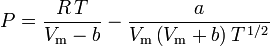$P = \frac{R\,T}{V_\mathrm{m} - b} - \frac{a}{V_\mathrm{m}\left(V_\mathrm {m} + b\right)T^{\,1/2}}$$a = \frac{R^2\, T_c^{\,5/2}}{2.3393\, P_c}$   and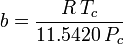$b = \frac{R\, T_c}{11.5420\, P_c}$$Z = \frac{V_\mathrm{m}}{V_\mathrm{m} - b} - \frac{a}{R\, T\left(V_\mathrm {m} + b\right)T^{\,1/2}}$
#####  Soave-Redlich-Kwong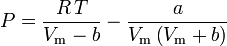$P = \frac{R\,T}{V_\mathrm{m} - b} - \frac{a}{V_\mathrm{m}\left(V_\mathrm {m} + b\right)}$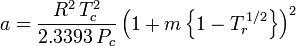$a = \frac{R^2\,T_c^2}{2.3393\,P_c}\, \Big(1 + m\, \Big\{1 - T_r^{\,1/2} \Big\} \Big)^2$   and$b = \frac{R\, T_c}{11.5420\, P_c}$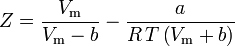$Z = \frac{V_\mathrm{m}}{V_\mathrm{m} - b} - \frac{a}{R\, T\left(V_\mathrm {m} + b\right)}$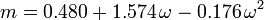$m = 0.480 + 1.574\, \omega - 0.176\, \omega^2$

The acentric factor, ω, used as a parameter in the Soave-Redlich-Kwong and the Peng-Robinson equations was first introduced by Pitzer et al in 1955. It can be expressed as:$\omega = \log_{10} \left(\frac{P_c}{P_\mathrm{vp}}\right) - 1$

where Pvp is the saturated vapor pressure of the gas at a temperature of$T = 0.7\, T_c$.

#####  Peng-Robinson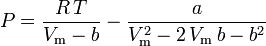$P = \frac{R\,T}{V_\mathrm{m} - b} - \frac{a}{V_\mathrm{m}^2 - 2\,V_\mathrm{m}\, b - b^2}$$a = \frac{R^2\,T_c^2}{2.1870\,P_c}\, \Big(1 + k\, \Big\{1 - T_r^{1/2} \Big\} \Big)^2$   and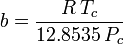$b = \frac{R\, T_c}{12.8535\, P_c}$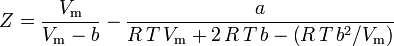$Z = \frac{V_\mathrm{m}}{V_\mathrm{m} - b} - \frac{a}{R\,T\,V_\mathrm{m} + 2\,R\,T\,b - \left(R\,T\, b^2/V_\mathrm{m}\right)}$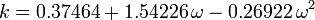$k = 0.37464 + 1.54226\, \omega - 0.26922\, \omega^2$

###  Virial equation of state

The most fundamental equation of state , in that it has the most sound theoretical foundation, is the virial equation of state: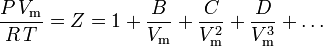$\frac{P\,V_\mathrm{m}}{R\,T} = Z = 1 + \frac{B}{V_\mathrm{m}} + \frac{C}{V_\mathrm{m}^2} + \frac{D}{V_\mathrm{m}^3} + \dots$

It was first proposed on an empirical basis by Thiesen in 1885 and further developed in 1901 by Kamerlingh-Onnes. A statistical-mechanical expression for B was first derived by Ornstein in his Ph.D Thesis. The equation was subsequently re-developed by H.D. Ursell in 1927 on a theoretical basis derived from a statistical-mechanical (cluster) analysis of intermolecular forces.

The parameters B, C and D are referred to as the second, third and fourth virial coefficients, respectively. The coefficients are not constants since they vary from one gas to another as well as the temperature of the gas under consideration. They are sometimes written as B(T), C(T) and D(T) to denote that they are functions of temperature. The numerical value of the coefficients must be determined experimentally.

The second virial coefficient provides the largest part of the correction for the non-ideal behavior of a gas. For that reason, the virial equation is sometimes truncated after the term containing the second coefficient. However, when the third coefficient is available, the equation is usually truncated after the term containing the third coefficient. The fourth coefficient is rarely available.

An extensive compilation of virial coefficients for pure gases and gas mixtures was prepared from the virial coefficient database at the Thermodynamics Research Center, formerly at Texas A&M University, College Station, Texas and now located at the National Institute of Standards and Technology (NIST), Boulder, Colorado. The latest version of that compilation was published in 2003. NIST also offers for purchase a software program, NIST Reference Fluid Thermodynamic and Transport Properties Database (REFPROP), that includes second and third virial coefficients as well as a host of other thermodynamic properties.

###  Other equations of state

There are a number of other equations of state for predicting the behavior of real gases. Perhaps, the best known are the Beattie-Bridgeman equation developed in 1929 and the Benedict-Rubin-Webb equation developed in 1940. In general, they are more complex than the equations discussed above.

###  Notation for the gas constant

The gas constant used in this article is the universal gas constant, R, that applies to any gas. There is also a specific gas constant, which can be denoted as Rs, and is defined as Rs = R / M where M is the molecular weight.

Unfortunately, many authors in the technical literature sometimes use R as the specific gas constant without denoting it as such or stating that it is the specific gas constant. This can and does lead to confusion.